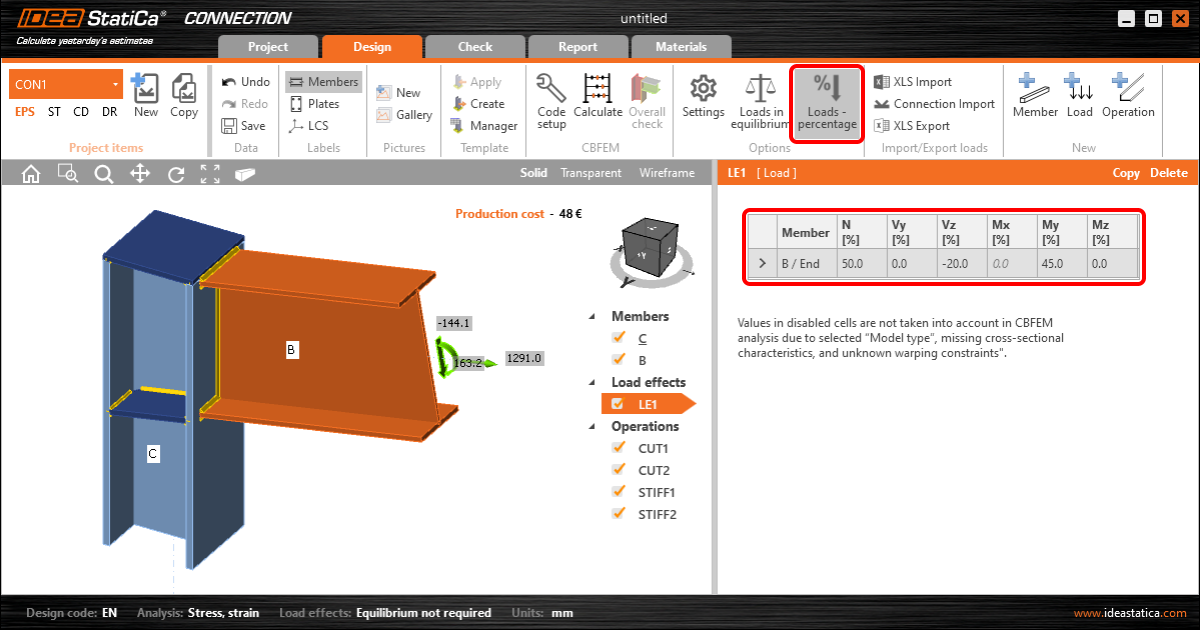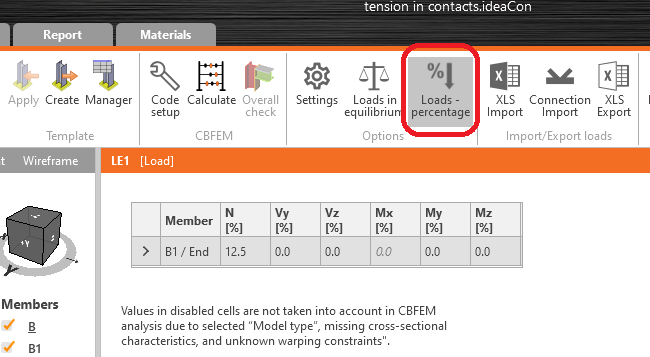### Choose language$$A new and simple option on how to set the load effects was added into IDEA StatiCa Connection 20.1. The members can be loaded by a defined percentage of the capacity of its cross-section. The setting of loads as a percentage of cross-section capacity is meant mainly as a simple tool. Setting loads in equilibrium is preferred.The cross-sectional properties can be viewed in the Materials tab. Torsion is disabled because the member resistance in torsion cannot be clearly determined due to unknown warping constraints.The internal forces for load effects are calculated as follows: Normal load: $$N=A \cdot f_y / \gamma_{M0}$$ Shear load: $$V_z =\frac{A_z \cdot f_y}{\sqrt{3} \cdot \gamma_{M0}}$$ $$V_y =\frac{A_y \cdot f_y}{\sqrt{3} \cdot \gamma_{M0}}$$ Bending moment: $$M_y = W_{el,y} \cdot f_y / \gamma_{M0}$$ $$M_z = W_{el,z} \cdot f_y / \gamma_{M0}$$ Note that $$\gamma_{M0}$$ is a material safety factor and its designation may be different among the codes. Also note that some codes, such as AISC, use slightly different values for shear loading, e.g. 0.6. However, in IDEA StatiCa Connection, the formulas follow von Mises yield criterion and are the same among all the codes. Available in Expert and Enhanced edition.$$